# Wolfram|Alpha Performs Vector Operations

June 1, 2011 —Comments Off

What do you get when you cross a mountain climber with a mosquito? Nothing—you can’t cross a scalar with a vector!

But what do you get when you cross two vectors? Wolfram|Alpha can tell you. For example: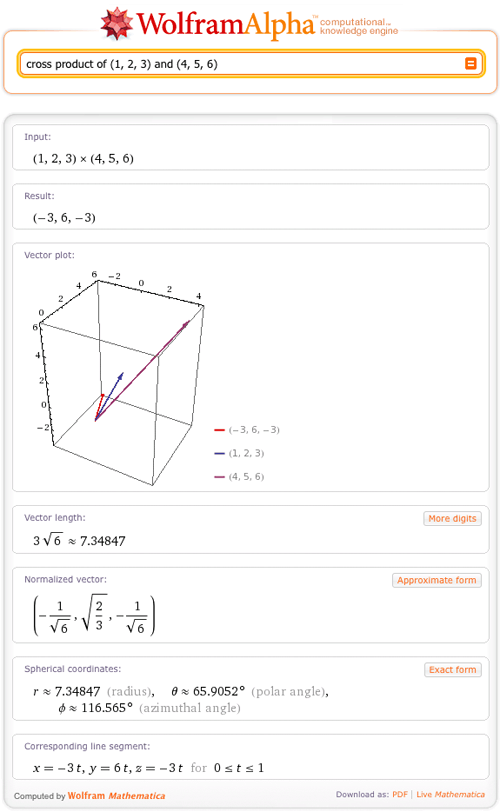And in fact, Wolfram|Alpha can give lots of information on vectors. A vector is commonly defined as a quantity with both magnitude and direction and is often represented as an arrow. The direction of the arrow matches the direction of the vector, while the length represents the magnitude of the vector. Wolfram|Alpha can now plot vectors with this arrow representation in 2D and 3D and return many other properties of the vector.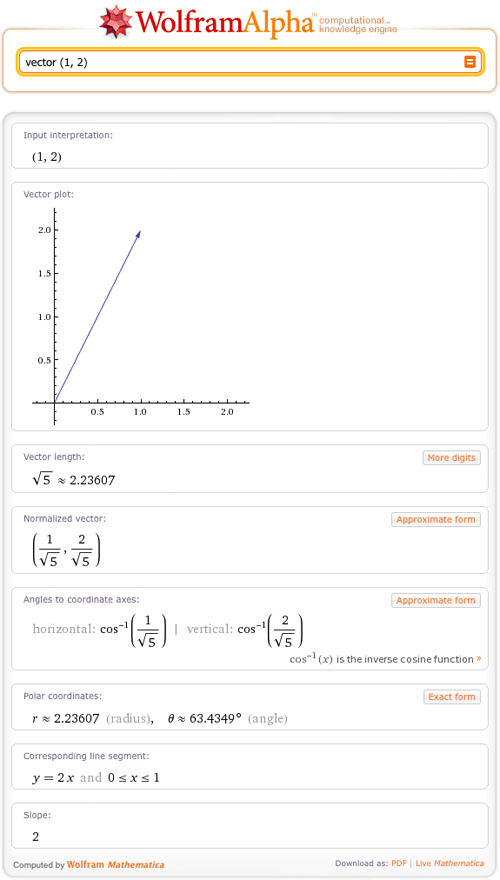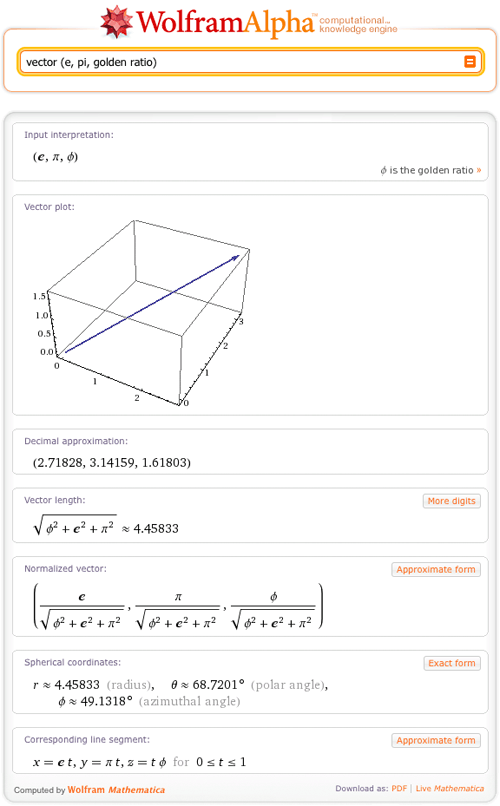Suppose you know only the point in R^n corresponding to your vector and you want to know its magnitude and direction. You can query Wolfram|Alpha for the vector’s length to find its magnitude: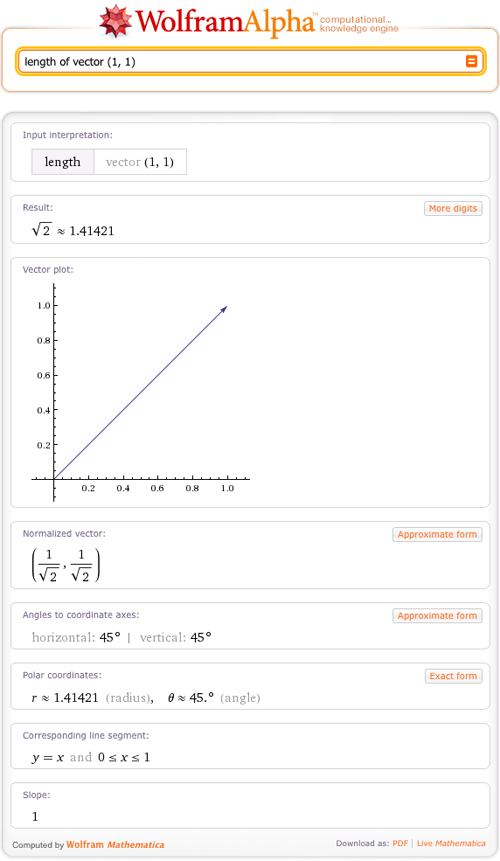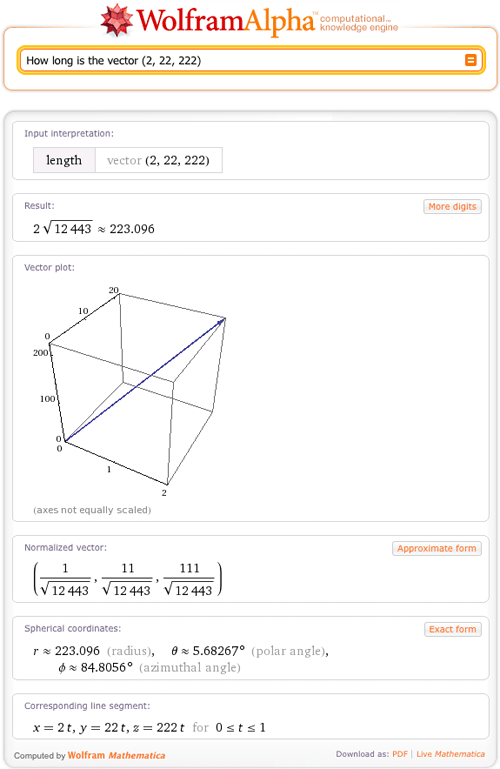And to find the direction, you can ask for the angles between the vector and the coordinate axes: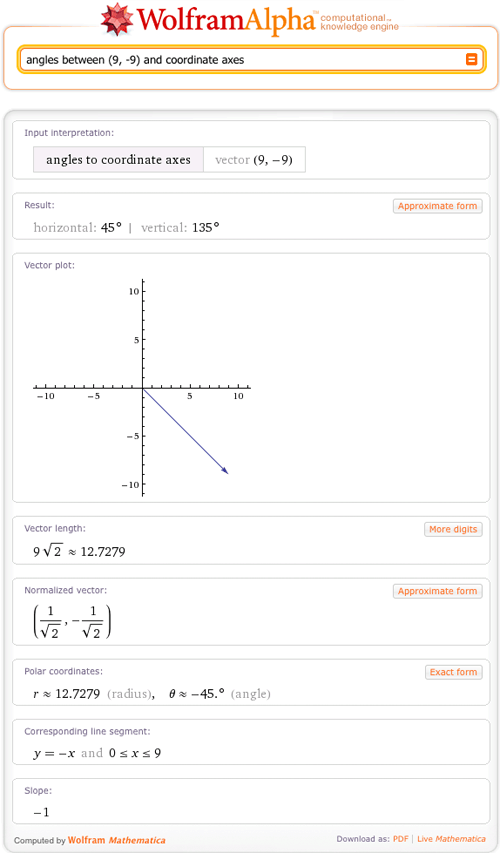If you want to find both the magnitude and direction, you can represent the vector in polar or spherical coordinates. The radius gives you the magnitude of your vector, while the angles specify its direction.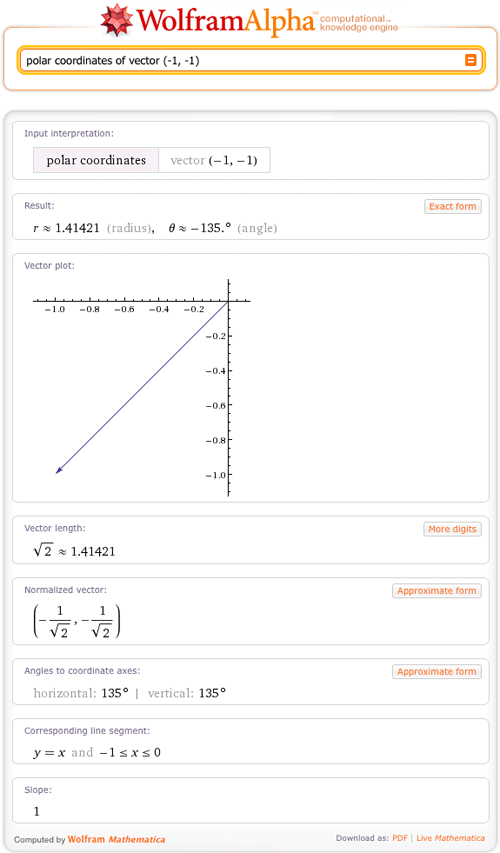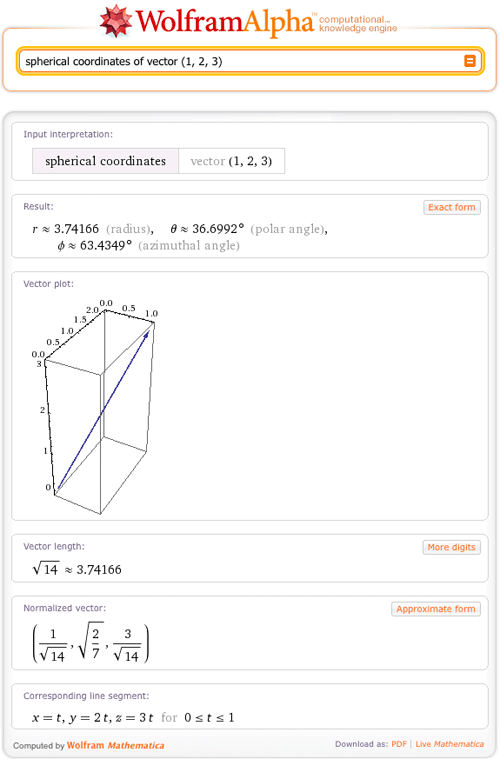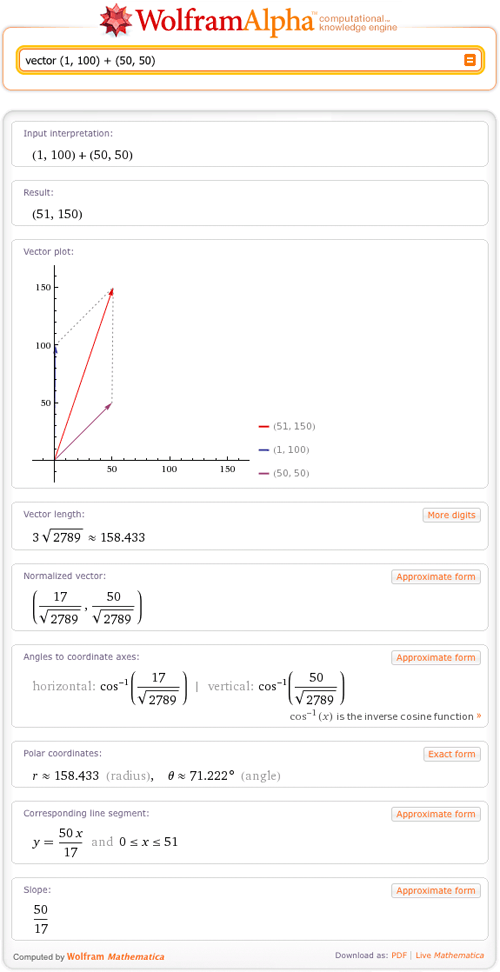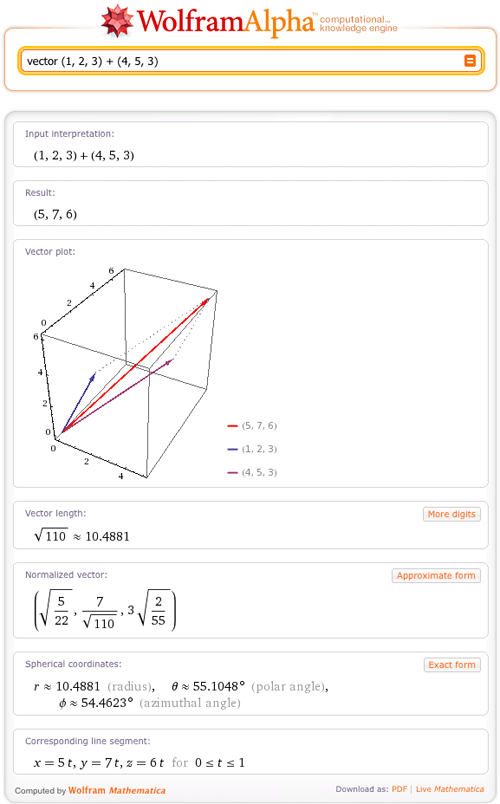So whether your studies are in algebra, calculus, or physics, Wolfram|Alpha can be your resource for learning about vectors.

Posted by J Januszewski June 1, 2011 at 3:38 pm

Lovely! Just asking: why do I keep getting error 101 when trying to compute something at W|A, and that I cannot use your calculus or W|A app?

Posted by Santiago Sinisterra June 1, 2011 at 5:54 pm

Are we ever going to have Mathematica for iPad?

Posted by Libby June 1, 2011 at 10:29 pm

This is excellent! Thanks…..I will definitely be playing with this new toy!

Posted by fizixx June 10, 2011 at 8:29 am